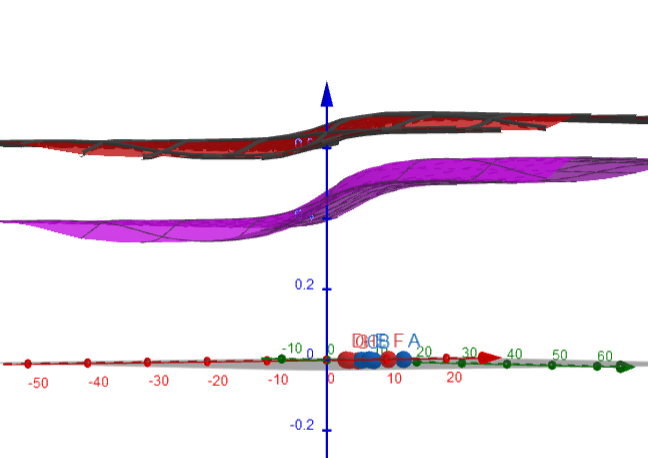# Fill area not bounded by linear equations

seegerer shared this question 3 years ago

Hi there,

for a visualization of a neural network within GeoGebra, I try to fill the area where the value of a function called gemuese is smaller than a value of the function called obst.

The network has 2 input neurons (x,y), 3 hidden neurons (h1,h2,h3), and 2 output neurons (gemuese, obst). If the output of gemuese (German for vegetable) is now bigger than the output for obst (German for fruit) the network decides predicts a vegetable.

This works fine if the sigmoid function is a linear function (to check, just change the sigmoid function to the identify function (sigmoid(a)=a) for a moment).

To achieve non-linearity I use the sigmoid function. The problem is, that the area stops being filled as soon as I use the sigmoid function (or any other non linear function (e.g. sigmoid(a)= a^2)).

Here is the corresponding GeoGebra project: https://www.geogebra.org/geometry/tgfhp3zn

And here is a working Desmos Version of what I try to acchieve: https://www.desmos.com/calc... . (The function gemuese is called g, the function obst is called o there).

Is there a way to recreate that same behaviour within GeoGebra?

Best,Stefan1

use x , y like variables of functions ever1

Thank you for your answer.If I interpret your answer correctly, I assume one way to do that would be to include sigmoid into obst(x,y) = 1/(1+e^((-1)* (...)) .Sadly, that does not work either.1

I put x in sigmoid(a), I copied your work and opened the 3d view in classic version and I did not see when obst()<=gemuesse() so GG does not show anything